## 5 Screenshots

•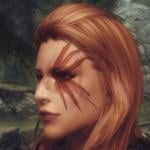•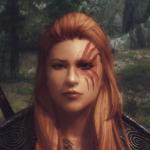•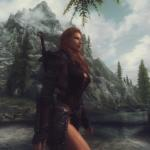•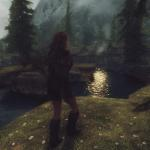•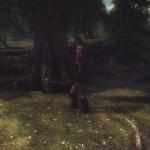Standalone female Nord Follower called Ysgrette Iron-Heart or an Aela replacer with nothing but her face and body edited. (Can't be used together)

Body: 7B TBBP

Voice Type: FemaleEvenToned

Class: CombatRanger

Level: Auto level 10-100

Essential: No

Protected: Yes

Perks: None (I think followers are too powerful with perks)

Location: See screenshots

Default Equipment: Ancient Nord Armour (No Helmet), Iron sword, Hunting Bow, 100 Iron Arrows.

=+=+=+=+=+=+=+=+=+=+=+=+=+=+=+=+=+=+=+=+=+=+=+=+=+=+=+=+=+=+=+=+=+=+=+=+=+=+=+=+=+=+=+=+=+=+=+=+=+=+=+=+=+=+=+=+=+=+=+=+=+=

Requirements

Patch 1.9 (The last patch)

XP32 Maximum Skeleton -XPMS by xp32

=+=+=+=+=+=+=+=+=+=+=+=+=+=+=+=+=+=+=+=+=+=+=+=+=+=+=+=+=+=+=+=+=+=+=+=+=+=+=+=+=+=+=+=+=+=+=+=+=+=+=+=+=+=+=+=+=+=+=+=+=+=

Installation

Manually: Copy and paste the meshes and textures folders and Ysgrette follower or Aela Replacer.esp into the Skyrim Data folder. Click "yes to all" if asked.

Or use Nexus Mod Manager.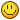NOTE: You can only use 1 of them. The Replacer and the Standalone follower use the same named .esp.

=+=+=+=+=+=+=+=+=+=+=+=+=+=+=+=+=+=+=+=+=+=+=+=+=+=+=+=+=+=+=+=+=+=+=+=+=+=+=+=+=+=+=+=+=+=+=+=+=+=+=+=+=+=+=+=+=+=+=+=+=+=

Uninstallation

If Ysgrette is following, Dismiss her then leave the cell (go through a loading screen).

Manually:

Data/meshes - then remove the folder named Ysgrette Iron-Heart

Data/meshes/actors/character/FaceGenData/FaceGeom - then remove the folder named Ysgrette follower or Aela replacer.esp

Data/textures - then remove the folder named Ysgrette Iron-Heart

Data/textures/actors/character/FaceGenData/FaceTint - then remove the folder named Ysgrette follower or Aela replacer.esp

Now remove Ysgrette Follower or Aela Replacer.esp from the Skyrim Data Folder.

=+=+=+=+=+=+=+=+=+=+=+=+=+=+=+=+=+=+=+=+=+=+=+=+=+=+=+=+=+=+=+=+=+=+=+=+=+=+=+=+=+=+=+=+=+=+=+=+=+=+=+=+=+=+=+=+=+=+=+=+=+=

Credits

Mature Skin by Maevan2

Fair Skin Complexion by HHaleyy

SG hair by HelloSanta

SG Female Eyebrows by Hello Santa

SG Female Textures Renewal by Hello Santa

Battle Hardened Warpaints by Diethardt and netherwalk

The Eyes Of Beauty by Gabriel Mailhot

Smile in HD by zzjay

High Resolution Scars by Xenius

SeveNBase a custom FemaleBodyReplacer by Crosscrusade DeMoNhUnTeR1986 Sevennity

Newsea (the original mesh author of hair used in the SG hair version) Newseasims.com

Robton for the Iron-Heart banner

And the testers for.... testing...

=+=+=+=+=+=+=+=+=+=+=+=+=+=+=+=+=+=+=+=+=+=+=+=+=+=+=+=+=+=+=+=+=+=+=+=+=+=+=+=+=+=+=+=+=+=+=+=+=+=+=+=+=+=+=+=+=+=+=+=+=+=

Recommended

Follower Commentary Overhaul - FCO by terzaerian

=+=+=+=+=+=+=+=+=+=+=+=+=+=+=+=+=+=+=+=+=+=+=+=+=+=+=+=+=+=+=+=+=+=+=+=+=+=+=+=+=+=+=+=+=+=+=+=+=+=+=+=+=+=+=+=+=+=+=+=+=+=